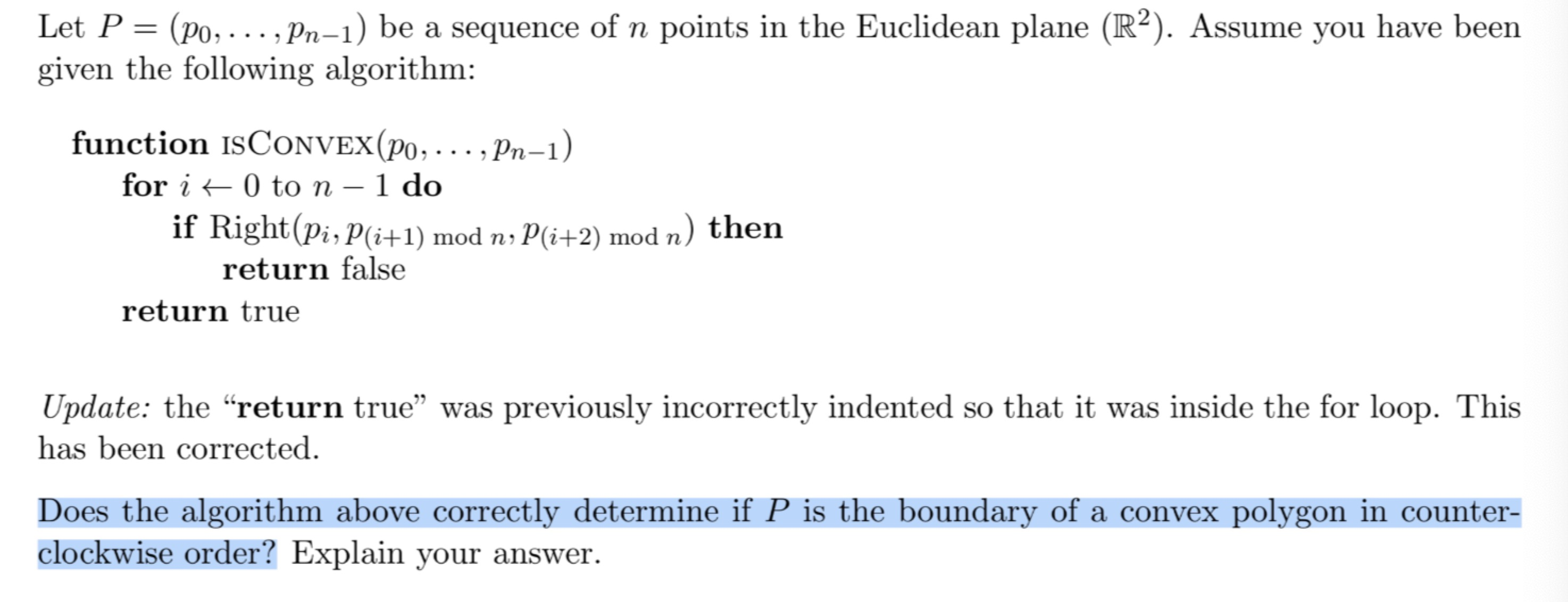2019-04-06 12:12

C 语言 检测点是否在凸边行内侧，学校算法作业一部分 检测是否是凸边行

P = （p0,.......pn-1）是一个有n个点的集合 属于 R^2。

Right应该是代入三点，检测三点位置是不是一个点在另外两点的右边• 写回答
• 好问题 提建议
• 追加酬金
• 关注问题
• 收藏
• 邀请回答

4条回答默认 最新

•huanyeliu 2019-04-07 13:22
已采纳

p(i+1) mod n 和 p(i+2)mod n是因为除了前n-2组点:(p(0),p(1),p(2)), (p(1),p(2),p(3)),...(p(n-3),p(n-2),p(n-1))以外, 我们依然要检测两组点（p(n-2),p(n-1),p(0)）(此时i=n-2, (i+1)mod n=n-1,（i+2)mod n=0)和（p(n-1),p(0),p(1))(此时i=n-1, (i+1)mod n=0, (i+2)mod n =1)的right函数返回值，从图形上看就是一个“环”首尾相衔接的部分也需要检测。如果不用mod, 那对这两组点的第一组当i=n-2时，则i+2=n,超过n-1， 第二组当i=n-1时，则i+1=n, i+2=n+1， 也都超过了n-1, 而下标超过n-1的点是不存在的。所以用mod就能把下标超过n-1的点转化成头端点，效果上就达到了检测首尾衔接部分的两组点。

但这个算法本身不正确，或者说不完整，在run isConvex()之前，先要对n-1个坐标点排序。一个排序规则可以是:先找出横坐标最小的点为p(0), 再计算其余各点与p(0)连线与x轴正向的夹角， 按夹角从小到大的顺序排序其余各点p(1)....p(n-1), 最后将排好序的n个点作为isConvex()的参数。

评论
解决 无用
打赏 举报